﻿ Application of Fuzzy Relation Equations to Student AssessmentPublications are Open
Access in this journal
Article Versions
Export Article
• Normal Style
• MLA Style
• APA Style
• Chicago Style
Research Article
Open Access Peer-reviewed

### Application of Fuzzy Relation Equations to Student Assessment

Michael Gr. Voskoglou
American Journal of Applied Mathematics and Statistics. 2018, 6(2), 67-71. DOI: 10.12691/ajams-6-2-5
Published online: May 02, 2018

### Abstract

Mathematical modelling appears today as a dynamic tool for teaching/learning mathematics, because it connects mathematics with real world applications and therefore increases the student interest on the subject. Fuzzy relation equations, which are obtained by the composition of binary fuzzy relations, are used in this work as a tool for evaluating student mathematical modelling skills. A classroom application and other suitable examples are also presented illustrating our results.

### 1. Introduction

The student assessment is a very important task of Education, because apart of being a social need and demand it helps the instructors in designing their future plans for a more effective teaching procedure. When the student performance is evaluated by numerical scores, the traditional method to assess the mean performance of a student class is the calculation of the mean value of those scores. However, either for reasons of more elasticity or for comforting the teacher’s existing uncertainty about the exact value of the numerical scores corresponding to each student’s performance, frequently in practice the assessment is made not by numerical scores but by linguistic grades, like excellent, very good, good, etc. This involves a degree of fuzziness and makes the calculation of the mean value of grades impossible.

A popular in such cases method for evaluating the overall performance of a student class is the calculation of the Grade Point Average (GPA) index (e.g. see 14, Chapter 6, p.125). GPA is a weighted average in which greater coefficients (weights) are assigned to the higher grades, which means that it reflects not the mean, but the quality performance of a student class.

In an effort to evaluate the mean student performance in such fuzzy assessment cases, we have used in earlier works tools from Fuzzy Logic. More explicitly, representing a student class as a fuzzy set in the set of the linguistic grades assessing the student performance, we calculated the existing in it probabilistic or possibilistic uncertainty (e.g. see Chapter 5 of 14). This method is based on the fact that the greater is the reduction of the uncertainty after a student activity (e.g. test, problem-solving, learning a new subject matter, etc.), the more is the new information obtained by the class and therefore the better is its performance. However, this method has two disadvantages: First it involves laborious calculations and second it can be used for comparing the mean performance of two different classes only under the assumption that they have been proved to have equivalent skills before the corresponding activity, a condition that does not hold always in practice. For this reason, in later works we have used Triangular and Trapezoidal Fuzzy Numbers for assessing the student mean performance (e.g. see Chapter 7 of 14), a method that has been proved to be easier and more accurate than the calculation of the uncertainty.

On the other hand, Subbotin et al. 11, based on a fuzzy model that we had developed in an earlier work 12 for studying the process of learning a subject matter in the classroom, they properly adapted the popular in fuzzy mathematics Center of Gravity defuzzification technique for evaluating the student quality performance. This model was later named as the Rectangular Fuzzy Assessment Model, while Subbotin and his collaborators developed also several variations of it by changing the shape of the graph of the corresponding membership function (e.g. see Chapter 6 of 14).

Here a new approach will be developed involving the use of Fuzzy Relation Equations (FRE) for studying the student Mathematical Modelling (MM) skills. The rest of the paper is formulated as follows: The second section contains the background from Fuzzy Binary Relations (FBR) and FRE which is necessary for the understanding of the paper. In the third section the model that uses FRE for studying the process of MM is developed, while in the fourth section a classroom application and other suitably chosen examples are presented illustrating the new model in practice. Finally, the fifth section is devoted to our conclusion and to some hints for future research on the subject.

### 2. Fuzzy Relation Equations

Let U be the universal set of the discourse. It is recalled that a Fuzzy Set (FS) A in U is a set of ordered pairs of the form A = {(x, m(x)): x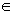U}, where m: U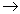[0, 1] is the membership function defining A. The value m(x) is called the membership degree of x in A. The closer is m(x) to 1, the better x satisfies the characteristic property of A. For example, if A is the FS of the old people of a city and m(x) = 0.7, then x is a rather old inhabitant of the city, etc. For general facts on fuzzy sets we refer to the book 5.

2.1 Definition: Let X, Y be two crisp sets. Then a FBR R(X, Y) is a FS on the Cartesian product X x Y of the form: R(X, Y) = {(r, mR(r): r = (x, y)X x Y}, where mR: X x Y [0, 1] is the corresponding membership function.

-When X = {x1,………,xn} and Y = {y1,……, ym}, then a FBR R(X, Y) can be represented by a n X m matrix of the form: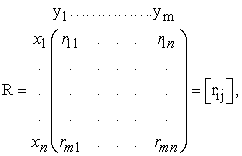where rij = mR (xi, yj), with i = 1,…, n and j =1,…, m. The matrix R is called the membership matrix of the fuzzy relation R(X, Y).

The basic ideas of fuzzy relations, which were introduced by Zadeh 15 and were further investigated by other researchers, are extensively covered in the book 4.

2.2 Definition: Consider two FBR P(X, Y) and Q(Y, Z) with a common set Y. Then, the standard composition of these relations, denoted by P(X, Y)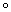Q(Y, Z) produces a FBR R(X, Z) with membership function mR defined by: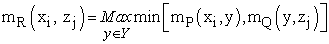(1)

for all i=1,…,n and all j=1,…,m. This composition is usually referred as max-min composition.

Compositions of FBR are conveniently performed in terms of the membership matrices of them. In fact, if P=[pik] and Q=[qkj] are the membership matrices of the FBR P(X, Y) and Q(Y, Z) respectively, then by equation (1) we get that the membership matrix of R(X, Y) = P(X, Y)Q(Y, Z) is the matrix R = [rij], with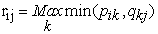(2)

2.3. Example: If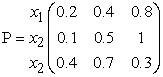and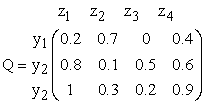are the membership matrices of the FBR P(X, Y) and Q(Y, Z) respectively, then by equation (2) the membership matrix of R(X, Z) is the matrix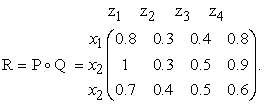Observe that the same elements of P and Q are used in the calculation of mR as would be used in the regular multiplication of matrices, but the product and sum operations are here replaced with the min and max operations respectively.

2.4 Definition: Consider the FBR P(X, Y), Q(Y, Z) and R(X, Z), defined on the sets, X = {xi: iNn}, Y = {yj : jNm}, Z= {zk : kNs}, where Nt = {1,2,…,t}, for t = n, m, k, and let P=[pij], Q=[qjk] and R=[rik] be the membership matrices of P(X, Y), Q(Y, Z) and R(X, Z) respectively. Assume that the above three relations constrain each other in such a way that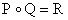(3)

wheredenotes the max-min composition. This means that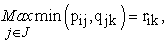(4)

for each i in Nn and each k in Ns. Therefore the matrix equation (3) encompasses nXs simultaneous equations of the form (4). When two of the components in each of the equations (4) are given and one is unknown, these equations are referred as FRE.

The notion of FRE was first proposed by Sanchez 10 and later was further investigated by other researchers ( 1, 2, 3, 6, 7, 8, 9, etc.).

### 3. A Study of MM Skills Using FRE

MM is a special type of mathematical solution of problems generated by corresponding real world situations. MM appears today as a dynamic tool for teaching/learning mathematics, because it connects mathematics with real world applications and therefore increases the student interest on the subject.

It is recalled that the process of MM involves the following steps: S1: Analysis of the problem, S2: Mathematization (formulation of the problem and construction of the model), S3: Solution of the model, S4: Validation (control) of the model and implementation of the final mathematical results to the real system. (for more details see 13). The validation and implementation of the model, which are usually considered as two different steps, have been joined, without loss of generality, in one step here, in order to make simpler the development of our new assessment model.

Let us now consider the crisp sets X = {M}, Y = {A, B, C, D, F} and Z = {S1, S2, S3, S4}, where M denotes the imaginary notion of the average student of a class and A = Excellent, B = Very Good, C = Good, D = Fair and F = Failed are the linguistic grades used for the assessment of the student performance.

Further, let n be the total number of students of a class and let ni be the numbers of students of the class who obtained the grade i assessing their performance, iY. Then one can represent the average student of the class as a FS on Y in the form M = {(i,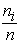): iY}.

The FS M induces a FBR P(X, Y) with membership matrix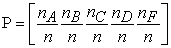In an analogous way the average student of a class can be represented as a FS on Z in the form M΄ = {(j, m(j): jZ}, where m: Z[0, 1] is the corresponding membership function. In this case the FS M΄ induces a FBR R(X, Z) with membership matrix R = [m(S1) m(S2) m(S3) m(S4)].

We consider also the FBR Q(Y, Z) with membership matrix the 5X4 matrix Q = [qij], where qij = mQ(i, j) with iY and jZ and the FRE encompassed by the matrix equation (3), i.e. by PQ = R.

When the matrix Q is fixed and the row-matrix P is known, then the equation (3) has always a unique solution with respect to R, which enables the representation of the average student of a class as a fuzzy set on the set of the steps of the MM process. This is useful for the instructor for designing his/her future teaching plans. On the contrary, when the matrices Q and R are known, then the equation (3) could have no solution or could have more than one solution with respect to P, which makes the corresponding situation more complicated.

### 4. A Classroom Application and Other Examples

The model presented in the previous section will be illustrated here with a classroom application and other suitable examples.

4.1. The Classroom Application

The following experiment took place at the Graduate Technological Educational Institute of Western Greece, in the city of Patras, when I was teaching to a group of 60 students of the School of Technological Applications (future engineers) the use of the derivative for the maximization and minimization of a function. A written test was performed after the end of the teaching process involving the two problems listed in the Appendix at the end of this paper. The results of the test are depicted in Table 1.

Therefore, the average student M of the class can be represented as a fuzzy set on Y = {A, B, C, D, F} by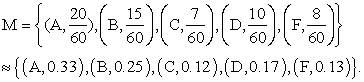Thus M induces a binary fuzzy relation P(X, Y), where X = {M}, with membership matrix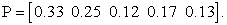Also, using statistical data of the last five academic years concerning the MM skills of the students of the School of Technological Applications of the Graduate Technological Educational Institute of Western Greece, we fixed the membership matrix Q of the binary fuzzy relation Q(Y, Z), where Z = {S1, S2, S3, S4}, in the form: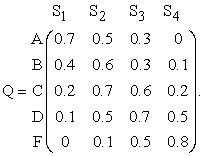The statistical data were collected by the instructor who was inspecting the student reactions during the solution of several MM problems in the classroom.

Next, using the max-min composition of FBR one finds that the membership matrix of R(X, Z) = P(X, Y) o Q (Y, Z) is equal to R = P o Q = [0.33 0.33 0.3 0.17].

Therefore the average student of the class can be expressed as a fuzzy set on Z by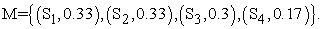The conclusions obtained from the above expression of M are the following:

• Only the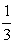of the students of the class were ready to use contents of their memory (background knowledge, etc.) in order to facilitate the solution of the given problems.

• All the above students were able to design the model and almost all of them were able to execute the solutions of the given problems.

• On the contrary, half of the above students did not succeed to check the correctness of the solutions found and therefore to implement correctly the mathematical results to the real system.

The first conclusion was not surprising at all, since the majority of the students have the wrong habit to start studying the material of their courses the last month before the final exams. On the other hand, the second conclusion shows that the instructor’s teaching procedure was successful enabling the diligent students to plan and execute successfully the solutions of the given problems. Finally, the last conclusion is due to the fact that students, when solving MM problems, they usually omit to check if their solutions are compatible to the restrictions imposed by the real world (for example see the two problems in the Appendix). Therefore, the instructor should emphasize during his/her lectures that the last step of the MM process (checking and implementation) is not a formality, but it has its own importance for preventing several mistakes.

Let us now consider the case where the membership matrices Q and R are known and we want to determine the matrix P representing the average student of the class as a fuzzy set on Y. This is a complicated case because we may have more than one solution or no solution at all. The following two examples illustrate this situation:

4.2 Example: Consider the membership matrices Q and R of the previous application and set P = [p1 p2 p3 p4 p5].

Then the matrix equation P o Q = R encompasses the following equations:

max {min (p1 , 0.7), min (p2, 0.4), min (p3, 0.2),

min (p4, 0.1), (p5, 0)}= 0.33

max {min (p1 , 0.5), min (p2, 0.6), min (p3, 0.7),

min (p4, 0.5), min (p5, 0.1)}= 0.33

max {min (p1 , 0.3), min (p2, 0.3), min (p3, 0.6),

min (p4, 0.7), (p5, 0.5)}= 0.3

max {min (p1 , 0), min (p2, 0.1), min (p3, 0.2), min (p4, 0.5),

min (p5, 0.8)}= 0.17

The first of the above equations is true if, and only if, p1 = 0.33 or p2 = 0.33, values that satisfy the second and third equations as well. Also, the fourth equation is true if, and only if, p3 = 0.17 or p4 = 0.17 or p5 = 0.17. Therefore, any combination of values of p1, p2, p3, p4, p5 in [0, 1] such that p1 = 0.33 or p2 = 0.33 and p3 = 0.17 or p4 = 0.17 or p5 = 0.17 is a solution of P o Q = R.

Let S(Q, R) = {P: P o Q = R } be the set of all solutions of P o Q = R. Then one can define a partial ordering on S(Q, R) by P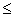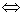pii,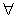ι = 1, 2, 3, 4, 5.

It is well established that whenever S(Q, R) is a non empty set, it always contains a unique maximum solution and it may contain several minimal solutions 11. It is further known that S(Q, R) is fully characterized by the maximum and minimal solutions in the sense that all its other elements are between the maximal and each of the minimal solutions 11. A method of determining the maximal and minimal solutions of P o Q = R with respect to P is developed in 2.

4.3 Example: Let Q = [qij], i = 1, 2, 3, 4, 5 and j = 1, 2, 3, 4 be as in Example 2 and let R = [1 0.33 0.3 0.17]. Then the first equation encompassed by the matrix equation P o Q = R is

max {min (p1 , 0.7), min (p2, 0.4), min (p3, 0.2),

min (p4, 0.1), min (p5, 0)}= 1.

In this case it is easy to observe that the above equation has no solution with respect to p1, p2, p3, p4, p5, therefore P o Q = R has no solution with respect to P.

In general, writing R = {r1 r2 r3 r4}, it becomes evident that we have no solution if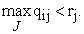### 5. Conclusion

In the present article we used FRE for assessing student MM skills. In this way we have managed to express the “average student” of a class as a fuzzy set on the set of the steps of the MM process, which gives valuable information to the instructor for designing his/her future teaching plans. On the contrary, we have realized that the problem of representing the “average student” of a class as a fuzzy set on the set of the linguistic grades characterizing his performance using FRE is complicated, since it may have more than one solutions or no solution at all.

In general, the use of FRE looks as being a powerful tool for the assessment of human skills and therefore our future research plans include the effort of using them in other human activities apart from the MM process, like learning, decision-making, etc.

### Appendix

The MM problems used in the Classroom Application of Section 4 are the following:

Problem 1: We want to construct a channel to run water by folding the two edges of a rectangle metallic leaf having sides of length 20 cm and 32 cm, in such a way that they will be perpendicular to the other parts of the leaf. Assuming that the flow of water is constant, how we can run the maximum possible quantity of the water through the channel?

Solution: Folding the two edges of the metallic leaf by length x across its longer side the vertical cut of the constructed channel forms an orthogonal with sides x and 32-2x (Figure 1).

View option
• Figure 1. The vertical cut of the channel

The area of the rectangle, which is equal to E(x) = x(32-2x) = 32x-2x2, has to be maximized. Taking the derivative E΄(x) the equation E΄(x) = 32-4x = 0 gives that x = 8cm. But E΄΄(x) = - 4 < 0, therefore E(8) = 128cm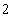is the maximum possible quantity of water to run through the channel.

Remark: A number of students folded the edges of the other side of the leaf and they found that E(x) = x(20-2x) = 20x-2x. In this case the equation E΄(x) = 0 gives that x = 5 cm, while E(5) = 50 cm. Their solution was mathematically correct, but many of them failed to realize that it is not acceptable in practice (real world)

Problem 2: Among all the cylindrical towers having a total surface of 180π m2, which one has the maximal volume?

Solution: Let R be the radius of the basement of the tower and let h be its height. Then its total surface is equal to 2πRh+2π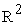=180π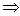h =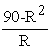. Therefore the volume of the tower as a function of R is equal to V(R) = π=90πR-π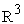. But V΄(R) = 90π-3π= 0 gives that R =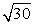m, while V΄΄(R) = -6πR < 0. Thus, the maximal volume of the tower is equal to V() =90π-π()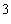= 60π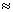1032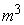Remark: A number of students considered the total surface of the tower as being equal to 2πRh, not including to it the areas of its basement and its roof. In this case they found that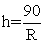, V(R) =90πR and V΄(R) = 90π >0, which means that under these conditions there is no tower having a maximal volume. However, some of these students failed to correct their model in order to find the existing solution of the real problem (unsuccessful transition from the model to the real world).

### References

  Czogala, E., Drewniak, J. & Pedryz, W., Fuzzy relation equations on a finite set, Fuzzy Sets and Systems, 7, 89-101, 1982. In article View Article  Higashi, M. & Klir, G.J., Resolution of finite fuzzy relation equations, Fuzzy Sets and Systems, 13, 65-82, 1984. In article View Article  Ghosh, D. & Pal, A., Analysis of Faculty Teaching Based on Student Feedback Using Fuzzy Relation Equations, International Journal of Applications of Fuzzy Sets and Artificial Intelligence, 5, 94-109, 2015. In article View Article  Kaufmann, A., Introduction to the Theory of Fuzzy Subsets, New York: Academic Press, 1975. In article  Klir, G. J. & Folger, T. A., Fuzzy Sets, Uncertainty and Information, New Jersey: Prentice-Hall, 1988. In article  Paul, L. C., Wang, Fuzzy relation equation (II): the branch- point solutions and the categorized minimal solutions, Springer, 2006. In article View Article  Perfilieva, I., Fuzzy function as an approximate solution to a system of fuzzy relation equations, Fuzzy Sets and Systems, 147 (3), 363-383, 2004 In article View Article  Perfilieva, I. Noskova, L., System of fuzzy relation equation with inf- composition: Complete set of solutions, Fuzzy Sets and Systems, 159 (17), 2256-2271, 2008. In article View Article  Prevot, M., Algorithm for the solution of fuzzy relations, Fuzzy Sets and Systems, 5, 319-322, 1981. In article View Article  Sanchez, E., Resolution of Composite Fuzzy Relation Equations, Information and Control, 30, 38-43, 1976. In article View Article  Subbotin, I., Badkoobehi, H. & Bilotskii, N.N., Application of Fuzzy Logic to Learning Assessment, Didactics of Mathematics: Problems and Investigations, 22, 38-41, 2004. In article View Article  Voskoglou, M.Gr., The Process of Learning Mathematics: A Fuzzy Set Approach, Didactics of Mathematics: Problems and Investigations, 10, 9-13, 1999. In article View Article  Voskoglou, M.Gr., Mathematical Modelling as a Teaching Method of Mathematics, Journal for Research in Innovative Teaching (National University, CA, USA), 8(1), 35-50, 2015. In article View Article  Voskoglou, M.Gr., Finite Markov Chain and Fuzzy Logic Assessment Models: Emerging Research and Opportunities, Columbia, SC: Createspace.com. – Amazon, 2017. In article  L.A. Zadeh, Similarity relations and fuzzy orderings, Information Sciences, 3, 177-200, 1971. In article View ArticleThis work is licensed under a Creative Commons Attribution 4.0 International License. To view a copy of this license, visit http://creativecommons.org/licenses/by/4.0/